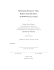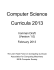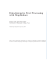# Efficient Distributed Linear Classification Algorithms via the

## Transcription

Efficient Distributed Linear Classification Algorithms via the
```Efficient Distributed Linear Classification Algorithms
via the Alternating Direction Method of Multipliers
Caoxie Zhang
Honglak Lee
Kang G. Shin
Department of EECS
University of Michigan
Ann Arbor, MI 48109, USA
Department of EECS
University of Michigan
Ann Arbor, MI 48109, USA
Department of EECS
University of Michigan
Ann Arbor, MI 48109, USA
[email protected]
[email protected]
[email protected]
Abstract
a single machine or a distributed system. Many algorithms for a single machine, such as LIBLINEAR 
and PEGASOS , have been developed and extensively used in both academia and industry. These algorithms can sequentially train on the entire data in a
few tens of rounds to obtain a good model and utilize
the sparsity of the data. However, training with largescale data on a single machine becomes slow because
of the disk I/O, not the CPU. Since the disk bandwidth is 2-3 orders of magnitude slower than memory bandwidth and CPU speed, much of the training
phase is spent on loading data rather than processing. This scenario worsens when the data cannot fit in
main memory, causing severe disk swaps. Yu et al. 
processing them in batches. Their algorithm reduced
the disk I/O time via pre-fetching and achieved a significant speedup over the original LIBLINEAR, which
is one of the state-of-art libraries for linear classification . However, since all single-machine algorithms
go through the entire data many times, they have to
load the same data from the disk multiple times when
the data cannot fit in memory. If the data requires
more than tens of gigabytes of storage (greater than
the typical RAM capacity), those algorithms are still
inefficient since the disk needs to be accessed more
than once and the training takes hours to complete.
Linear classification has demonstrated success in many areas of applications. Modern
algorithms for linear classification can train
reasonably good models while going through
the data in only tens of rounds. However,
large data often does not fit in the memory of
a single machine, which makes the bottleneck
in large-scale learning the disk I/O, not the
CPU. Following this observation, Yu et al.
(2010) made significant progress in reducing
disk usage, and their algorithms now outperform LIBLINEAR. In this paper, rather than
optimizing algorithms on a single machine,
we propose and implement distributed algorithms that achieve parallel disk loading and
access the disk only once. Our large-scale
learning algorithms are based on the framework of alternating direction methods of multipliers. The framework derives a subproblem
that remains to be solved efficiently for which
we propose using dual coordinate descent and
trust region Newton method. Our experimental evaluations on large datasets demonstrate that the proposed algorithms achieve
significant speedup over the classifier proposed by Yu et al. running on a single machine. Our algorithms are faster than existing distributed solvers, such as Zinkevich et
al. (2010)’s parallel stochastic gradient descent and Vowpal Wabbit.
1
Introduction
Large-scale linear classification has proven successful
in many areas, such as machine learning, data mining, computer vision, and security. With the burgeoning of social media, which provides an unprecedented
amount of user-provided supervised information, there
will likely be more extreme-scale data requiring classification. Training on these large data can be done on
Appearing in Proceedings of the 15th International Conference on Artificial Intelligence and Statistics (AISTATS)
2012, La Palma, Canary Islands. Volume XX of JMLR:
W&CP XX. Copyright 2012 by the authors.
1398
On the other hand, distributed systems composed of
commercial servers (nodes) are becoming more prevalent both in research labs and medium-scale companies. A large Internet company may have thousands of
nodes. Algorithms running on distributed systems can
now load the data in parallel to the distributed memory and train without using disk any more, thereby significantly reducing the cumbersome disk loading time.
One important challenge in the field is to design new
algorithms in the parallel setting, since the current linear classification algorithms are inherently sequential.
In this paper, we propose distributed algorithms for
linear classification. The system architecture is illustrated in Fig. 1. We use the alternating direction
method of multipliers (ADMM) as the framework for
solving distributed convex optimization; see Boyd et
al.  for a review of ADMM. This framework introduces additional variables to regularize the difference
Caoxie Zhang, Honglak Lee, Kang G. Shin
Data distributed
in machines
Solving subproblems
in parallel
framework for solving distributed optimization. The
first work on ADMM may date back to the 1970s 
and most of the theoretical results have been established in the 1990s . However, until recently, ADMM
was not widely known in the field. For completeness,
we provide a review of its application to linear classification.
Aggregation and
# of features
Data x𝐵1
Data x𝐵2
x 𝐵1 , z
w1 , u1
x 𝐵2 , z
w2 , u2
⋮
Data x𝐵𝑚
𝐰+𝐮
𝐳
Given a dataset {(xi , yi )}li=1 (xi ∈ Rn , yi ∈ {−1, +1}),
we consider L2-regularized L2-loss (squared hinge loss)
SVM as the linear classification model. Our algorithms
also apply to hinge loss and squared loss as we show
in Sec. 5. Here, we focus on L2-loss for the sake of
presentation.
⋮
x 𝐵𝑚 , 𝐳
𝐰𝑚 , 𝐮𝑚
Figure 1: An illustration of the distributed linear classi-
fication algorithm. The data is split by instances across
machines. Each machine loads the local data in parallel
and keeps it in the main memory. Then each machine uses
efficient algorithms (e.g., Algorithm 1 in Sec. 3.1) to solve
a subproblem with the input being the local data and a
shared vector z to generate the output of a weight vector
and an auxiliary vector u. After solving the subproblems,
all machines aggregate these two vectors using averaging to
generate z and broadcast it for the next round of iterations.
among the models solved by the distributed machines.
The ADMM framework provides the freedom to propose efficient methods for solving the subproblems in
distributed machines. We propose a dual coordinate
descent method that achieves linear run-time complexity in the number of samples and takes advantage of
the feature vector’s sparsity. We also use the trust region Newton method to handle the dense data matrix.
l
min f1 (w) =
w
X
1
||w||22 + C
max(1 − yi wT xi , 0)2 , (1)
2
i=1
where C is a hyperparameter. For simplicity, we ignore
the bias term, although one can append a constant to
the feature vector. To make the problem amenable to
decomposition, we first let {B1 , . . . , Bm } be a partition
of all data indices {1, . . . , l}. Then, we write down an
equivalent problem as follows:
min
w1 ,...,wm ,z
m X
X
1
||z||22 + C
max(1 − yi wTj xi , 0)2
2
j=1
i∈Bj
+
m
X
j=1
Early work on distributed algorithms for kernel SVM
was done by Chang et al. , who used an approximate matrix factorization to solve the convex optimization. However, the run-time complexity of kernel
SVMs is at least quadratic in the number of samples.
The authors in [2, 7] used the ADMM framework to
solve a SVM problem in a single machine. However,
they used a general convex optimization method for
the subproblem, which has super linear complexity in
the number of samples; moreover, they did not evaluate performance on large data in realistic distributed
environments.
Our contributions are as follows. First, we propose
an efficient distributed linear classification algorithm
and space complexity. Second, our experimental evaluations on large datasets in distributed environments
show that the proposed approach is significantly faster
than other existing distributed approaches, such as (i)
parallel stochastic gradient descent using averaging to
aggregate solutions as proposed by Zinkevich et al. 
and (ii) Vowpal Wabbit (VW) . Our proposed algorithm is also significantly faster than single-machine
based algorithms, such as Block LIBLINEAR .
2
A Distributed Framework for Linear
Classification
In this section, we apply ADMM to linear classification
to yield the distributed algorithm. ADMM is a general
1399
ρ
||wj − z||22 ,
2
subject to wj − z = 0,
j = 1, . . . , m,
(2)
where ρ is a constant step size for later iterations. Here
we introduce a new weight vector wj that is associated
with
Bj , and a regularization vector z. The term
Pm data
ρ
2
||w
j − z||2 helps the algorithm converge more
j=1 2
robustly.
Let us denote w := {w1 , . . . , wm } and λ :=
{λ1 , . . . , λm }, λj ∈ Rn , j = 1, . . . , m. We can have
the Lagrangian of problem (2) as follows:
L(w, z, λ) =
m X
X
1
||z||22 + C
max(1 − yi wTj xi , 0)2
2
j=1
i∈Bj
+
m X
ρ
j=1
2
||wj − z||22 + λTj (wj − z) , (3)
where λ are the dual variables. ADMM consists of the
following iterations:
wk+1 = arg min L(w, zk , λk )
(4)
zk+1 = arg min L(wk+1 , z, λk )
(5)
w
z
λk+1
= λkj + ρ(wk+1
− zk+1 ),
j
j
j = 1, . . . , m.
(6)
Since the Lagrangian L is separable in wj , we can solve
Caoxie Zhang, Honglak Lee, Kang G. Shin
problem (4) in parallel:
wk+1
j
= arg min C
w0
X
T
i∈Bj
max(1 − yi w0 xi , 0)2 (7)
ρ
+ ||w0 − z||22 + λTj (w0 − z), j = 1, ..., m.
2
Also, zk+1 has a closed form solution:
z
k+1
=
ρ
Pm
j=1
wk+1
+
j
mρ + 1
Pm
j=1
λkj
.
(8)
3
A simple change of variables, letting λj = ρuj , can
make the quadratic form of wj in Eq. (8) more compact. We now have the new ADMM iterations as:
X
max(1 − yi wT xi , 0)2 (9)
wk+1
= arg min C
j
w
zk+1
uk+1
j
i∈Bj
ρ
+ ||w − zk + ukj ||22 , j = 1, . . . , m.
2
Pm
k+1
+ ukj
j=1 wj
=
m + 1/ρ
= ukj + wk+1
− zk+1 ,
j
high accuracy; however, our empirical experience suggests that ADMM usually takes only tens of iterations to achieve good accuracy. This few number of
the iterations can be enough for large-scale classification tasks since the SVM (or logistic regression) uses
loss functions that approximate the misclassification
errors, and it may not be necessary to minimize these
objectives exactly [1, 17]. In our experiments, we show
that our algorithm achieves fast convergence in training optimality and test accuracy within only a few tens
of ADMM iterations for large-scale datasets.
(10)
j = 1, . . . , m. (11)
Here, each machine j solves the subproblem (9) in parallel, which is only associated with data xBj , {xi :
i ∈ Bj }. Machine j also loads the data xBj from the
disk only once and stores them in the memory in the
ADMM iterations. Each machine only needs to communicate wj and uj without passing the data.
The ADMM iterations also give the following theoretical guarantees. For any ρ > 0 we have:
1. If we define the residual variable rk = [wk1 −
zk , . . . , wkm − zk ], then rk → 0 as k → ∞.
2. The objective function in problem (2) converges
to the optimal objective function of problem (1).
Although ADMM provides a parallel framework, the
issue of solving the subproblem (9) efficiently still remains. In this subproblem, each machine contains
a portion of the data, which can still be quite large
to solve. In this section, we present both a dual
method and a primal method. The dual method is
a coordinate descent method similar to the one in
. To obtain an -optimal dual solution, our method
has a computational complexity of O(nnz log(1/)),
where nnz is the total number of non-zero features
in the data. For well-scaled data, the term log(1/)
(including the constant) usually becomes a few of
tens to achieve good accuracy, namely, the algorithm
only needs to sequentially pass the data in a few
of tens rounds. The primal method is a trust region Newton method similar to the one in . This
method obtains an approximated Hessian using the
is O(nnz × number of conjugate gradient iterations ×
number of Newton iterations). The primal method
can be more efficient if the data matrix is dense. We
first detail the dual coordinate descent method and
then briefly describe the trust region Newton method.
3.1 A Dual Coordinate Descent Method
We rewrite the subproblem (9) in a more readable way:
s
min f2 (w) =
w
1
2
2 ||z||2
To show the above, since
and max(1 −
yi wT xi , 0)2 are both closed and proper convex functions and problem (1) possesses strong duality, they
meet the conditions to guarantee the convergence results . These results imply that eventually wkj would
agree upon a consensus vector zk , which would converge to the solution of the original SVM problem (1).
The auxiliary variables ukj convey to machine j how
different its wkj is from zk and serve as the signal to
pull wk+1
into consensus when machine j solves the
j
subproblem (9).
solves the dual problem of (2). However, it has a
better convergence result as compared to other distributed approaches, such as the dual decomposition
method . ADMM is a first order method, so it would
seem that it would take many iterations to achieve
1400
Efficient Algorithms for Solving the
Subproblem
X
ρ
||w − v||22 + C
max(1 − yi wT xi , 0)2 ,
2
i=1
(12)
where v = zk − ukj at the k-th ADMM iterations, and
{x1 , . . . , xs } denotes the data in xBj for some machine
j. The above problem is different from traditional
SVM in its regularization term, which also considers
the consensus to other machines’ solutions. Therefore,
we still need an efficient special solver for this problem.
The dual problem of (12) can be written as a quadratic
programming:
1 T
α Q̄α − bT α
2ρ
subject to αi ≥ 0, ∀i,
min f3 (α) =
α
(13)
where Q̄ = Q + D, Qij = yi yj xTi xj , D is a diagonal
matrix, Dii = ρ/(2C) and b = [1 − y1 vT x1 , . . . , 1 −
ys vT xs ]T .
Caoxie Zhang, Honglak Lee, Kang G. Shin
Algorithm 1 A dual coordinate descent method for
solving the problem (12)
Ps
(0)
Initialize α(0) , w(0) = i=1 yi αi xi + v and t = 0.
(t)
while α is not optimal do
for i = 1 . . . s do
t=t+1
T
(t)
(t)
Gi = yi(
w(t) xi − 1 + Dii αi
We solve this problem using dual coordinate descent,
which optimizes one variable in α at a time and then
circularly moves to the next variable and so on. For
problem (13), the one-variable optimization has a close
form solution since it is a quadratic minimization. In
other words, for any i, we can optimize αi while fixing
(t)
other variables. Let Gi be the partial derivative of f3
with respect of αi at the t-th iteration, then we have
(t)
Gi =
(t)
αj Q̄ij − bi .
j=1
P Gi =
(14)
(t)
(t)
We can also use the projected partial derivative, de(t)
noted as P Gi , to determine the stopping criteria of
coordinate descent:
(
(t)
(t)
min(0, Gi ) if αi = 0,
(t)
P Gi =
(16)
(t)
Gi
otherwise.
(t)
(t)
If P Gi = 0, we do not need to update αi .
(t)
To get Gi in Eq. (14), the main computation is O(s).
However, due to the special structure of the problem,
we can reduce to O(n̄), where n̄ is the average number
of non-zero features in the data, to make the computational complexity independent of the size of the data,
s. The key idea here is to maintain an intermediate
vector w(t) at each iteration as
s
X
(t)
yj αj xj + v.
3.2 A Trust Region Newton Method
We use the trust region Newton method in  to solve
the problem (12). Since the Hessian in L2 loss SVM
does not exist, the authors in  used a generalized
Hessian for approximation. With the generalized Hessian they use the conjugate gradient method to find the
Newton-like direction and use the trust region Newton
method to iterate. The method is general and can be
directly applied to our problem (12). The only important difference is the gradient of the objective function:
X
X
∇f2 (w) = (ρ + 2C
xi xTi )w − 2C
yi xi − ρv, (20)
(17)
(t)
(t)
Gi
(t) T
= yi w
i∈I
as
xi − 1 +
(t)
Dii αi
(18)
The main computation is then the dot product of w(t)
and xi , which is O(n̄) if we save xi as a sparse form. To
(t)
(t+1)
maintain w(t) after αi changes to αi
, we update
it as follows:
(t+1)
(t)
w(t+1) = w(t) + αi
− αi yi xi .
(19)
This operation also takes O(n̄). The overall procedure
is provided in Algorithm 1.
For theoretical results, we can easily apply results in
 to show that Algorithm 1 converges and it takes
O(log(1/)) iterations of while-loops to achieve an accurate solution, i.e., f3 (α) ≤ f3 (α∗ ) + . Therefore, the total computation is O(sn̄ log(1/)) for an
-accurate solution, where sn̄ is the total number of
non-zero features in data xBj .
1401
i∈I
where I = {i|(1 − yi w(t) xi ) > 0}.
4
j=1
Then, we can express Gi
(t)
min(0, Gi ) if αi = 0,
(t)
Gi
otherwise.
if |P Gi | =
6 0 then
(t+1)
(t)
(t)
αi
= max αi − Gi /Qii , 0
(t+1)
(t)
w(t+1) = w(t) + αi
− αi yi xi
else
α(t+1) = α(t)
w(t+1) = w(t)
end if
end for
end while
Thus, the optimal αi will be the root of Gi projected
on [0, ∞). We can update αi as
!
P
(t)
bi − j6=i αj Q̄ij
(t+1)
,0
αi
= max
Q̄ii
(t)
(t)
= max αi − Gi /Q̄ii , 0
(15)
w(t) =
(t)
(t)
s
X
Improving the Distributed
Algorithms
We have discussed the use of two efficient methods
to solve the subproblem (9) under the framework of
ADMM. However, there is still room for further improvement of the distributed algorithms. In this section, we describe several simple but important techniques that can significantly affect efficiency.
Random permutation of data. Since each machine
processes only a portion of the data and communicates its solutions to other machines, if the local data
contains mostly the same label, it may take a large
number of ADMM iterations to achieve consensus. A
useful technique is to randomly shuffle the data to different machines to ensure that class labels in the data
are balanced. This technique reduces ADMM’s total
number of iterations.
Warm start in solving subproblem (9). In solving
subproblem (9), it is not necessary to start from some
fixed (e.g., zero) vector. In particular, wkj may not
change much at the very end of the ADMM iterations.
Caoxie Zhang, Honglak Lee, Kang G. Shin
So, we use wk−1 as the starting point for obtaining
wk . Specifically, in Algorithm 1 we save the previous
α used for obtaining wk−1 and reuse it as α0 . For
the primal method, we can directly use wk−1 as the
starting point for Newton iteration. This technique
shortens the time within each ADMM iteration.
Inexact minimization (early stopping) of subproblem (9). Solving (9) exactly, especially at the
initial ADMM iterations, may not be worthwhile since
the exact solutions usually require a large amount of
time and might not be the direction for achieving good
consensus. In fact, the problem (9) can be solved approximately. In Algorithm 1, we can limit the whileloop’s maximum number of iterations to be, for example, M times. This corresponds to going through the
local data only M rounds. In the trust region Newton
method, we can limit the number of Newton iterations.
This technique can dramatically speed up the ADMM
iterations.
Over-relaxation. wk+1
is used for updating z and
j
u in (10)–(11). In fact, wk+1
j
k
previous value of z to improve convergence. The new
b k+1
w
in (10)-(11) can be written as:
j
b k+1
w
= βwk+1
+ (1 − β)zk .
j
j
(21)
We used β ∈ [1.5, 1.8], as reported in . This technique can reduce the total number of ADMM iterations while achieving the same accuracy.
5
Implementations
Our algorithms are simple and easy to implement on
distributed systems. First, we consider their implementations from a data perspective. The data is split
uniformly by data instances for processing on different
machines. At the start, each machine loads its own
data in parallel to fit in its RAM. Data in memory is
represented as a sparse matrix, so the space complexity is O(|Bj |n̄) for machine j. Each machine j maintains its own wj and uj in its memory and processes
them in w-update and u-update in (9, 11) in parallel
with other machines. Machine j broadcasts wk+1
+ ukj
j
Pm
k+1
and waits to collect j=1 (wj + ukj ) for z-update in
(10). For the communication of wk+1
+ uk , and synj
Pm j k+1
chronization, i.e., waiting to collect j=1 (wj + ukj ),
we use the Message-Passing Interface (MPI)  for
inter-machine coordination, which is one of the most
popular parallel programming frameworks for scientific
applications. MPI supports high-level message communication and eliminates most of the programming
burdens for low-level synchronization.
Second, we also implement our algorithms for different loss functions such as hinge loss and square loss.
These loss functions would yield different forms of subproblem (9). However, we can apply the same idea
of dual coordinate descent to solving the subproblem
since it is still a quadratic programming and allows
us to use the sparsity trick of Eq. (17). We implemented all the algorithms, e.g., ADMM and subprob1402
lem solvers, in C/C++. Specifically, we use OpenMPI
for the communication in ADMM, and also modify the
LIBLINEAR library for solving the problem (9) using
the dual and primal methods. To further improve the
implementations, we also used the following additional
techniques.
Distributed normalization and evaluation of
test accuracy. Our empirical findings suggest that
normalizing the feature vector to unit length can be
very helpful to the convergence of classification algorithms. Therefore, when each machine loads the data,
it can normalize the data in parallel with other machines. Moreover, we can also evaluate the test data
in a distributed fashion if test data is too big to load
in one machine. These simple ideas allow the experiments to be done more efficiently.
Cross-validation and multiclass classification.
Cross-validation (hyperparameter selection) is easily
carried out in our implementations. Each machine
can separate its own data into training and validation sets and perform both training and validation in
a distributed fashion. For multiclass classification, we
implement a one-versus-the-rest (OVR) method, since
its accuracy is comparable to other surrogate-loss multiclass classifications [15, 10]. The OVR has essentially
N binary classifications, where N is the number of
classes. Note that, in our algorithms, the N binary
classifications need to load the data only once.
6
Experiments
In this section, we first show that our proposed algorithms are faster than other existing distributed approaches on four large datasets. We then demonstrate
a significant improvement over a single-machine solver
and provide an analysis on these gains.
We consider four large datasets: a document dataset
(webspam), an education dataset (kddcup10), and two
synthetic datasets (epsilon and kappa).1 The dataset
kappa is generated as follows: xi and w are uniformly
sampled from [−1, 1]n ; yi = sgn(wT xi ) and yi will flip
its sign with probability 0.1. Finally, we normalize
xi such that ||xi ||22 = 1. All datasets are split into
training and test sets with an 8:2 ratio, except the
kddcup10 dataset, which has already been separated
into training and test sets. We also use five-fold cross
validation to choose the best hyperparameter C since
we need to compare the accuracy to VW, which has
a different optimization problem. The details of the
datasets are summarized in Table 1.
Our distributed algorithms are evaluated by running
on 8 machines. Each machine has an Intel Core i7-950
processor (at 3.06GHz) and 12 GB RAM. The training/testing data is split and distributed evenly across
these nodes. The disk throughput is ∼140 MB/sec.
The machines are connected in a star network through
1
The first three datasets are available at http:
//www.csie.ntu.edu.tw/cjlin/libsvmtools/datasets/.
For kddcup10, we used a pre-possessed version of
bridge to algebra 2008 2009 in KDD Cup 2010 such that
each feature vector has unit norm.
Caoxie Zhang, Honglak Lee, Kang G. Shin
Table 1: Summary of the datasets. l is the number of examples, and n the number of features. We also show the total
number of non-zero features in the dataset. The memory represents the actual size of the data. Each element in the data
is represented as 4 bytes for index and 8 bytes for values. A 64-bit machine will align the data structure and cause each
element to have 16 bytes. Split is the initial time to split and compress the datasets into files. Spread is the initial time
for our algorithms to disseminate the files to the corresponding machines.
Dataset
l
n
# nonzeros Data size (GB)
webspam
350,000 16,609,143 1,304,697,446
20.9
32
881
26
kddcup10 20,012,498 29,890,095
588,310,963
9.4
1
712
148
epsilon
500,000
2,000 1,000,000,000
16
2
504
12
500,000
8,000 4,000,000,000
64 0.5
3913
299
kappa
a 1 Gigabit Ethernet with the TCP throughput of
∼111 MB/sec between any two machines.
6.1
Comparison with Other Distributed
Solvers
First, we compare our algorithms with three distributed solvers that can load data in parallel and access the disk only once. The first solver is an extended
version of Block LIBLINEAR . We set up the serialized version of linear classification to run on multiple
machines. All machines load the local data in parallel. Then only one machine runs at a time using Block
LIBLINEAR, passing the processed model to the next
machine as the initial model in a round-robin manner.
but leaves all machines idle except one during training.
The second solver uses parallel stochastic gradient descent (SGD), similar to Zinkevich et al. . The original algorithm sequentially passes the data only once
using SGD, and then aggregates an average model.
Here, we extend the algorithm by repeating such a
procedure: the averaged weight vector is used as the
initial point for the next round of SGD.
Wabbit (VW).2 VW implements a fast online learning
algorithm using SGD without regularization. The current version 6.0 starts to support running on clusters
by using similar ideas in parallel SGD. VW directly
uses sockets for the communications and synchronization of nodes. We would like to compare our method
and therefore might be faster than the second solver.
Now we describe the algorithms’ settings. Unless explicitly indicated, the loss functions are all squared
hinge loss. We used four different settings for our algorithms based on ADMM:
• DUAL: This uses the dual coordinate descent
method, i.e., Algorithm 1 to solve the subproblem (9). The stopping criterion for Algorithm 1
is maxi |P Gi | < 0.001, where all P Gi are in the
same for-loop in Algorithm 1.
• DUAL.M1: This is similar to DUAL, except that
it goes through the data only once in solving (9).
• PRIMAL: This uses the trust region Newton
method we described in Sec. 3.2. Its stopping criterion is that the norm of gradient is less than
0.01.
2
https://github.com/JohnLangford/vowpal_wabbit
1403
• PRIMAL.M1: This is similar to PRIMAL, but it
uses only one Newton step.
For ADMM iterations, we use over-relaxation with β =
1.6 and step size ρ = 1 for all cases.
Experimental settings for other distributed solvers are:
• D-B-LIBLINEAR: This uses distributed systems
to run the serialized Block LIBLINEAR.
• D-B-LIBLINEAR.M1: This is similar to D-BLIBLINEAR, except that each node only passes
through the data only once in each run.
• PSGD: Parallel stochastic gradient descent as in
Zinkevich et al. . We compute the aggregated
model every time the algorithm passes the whole
data. The learning rate is set to 10−3 as in .
• PSGD.D[x]: Rather than using a constant learning rate in the SGD updates of PSGD, it uses a
decaying learning rate where η(t) = x/(t + 1). We
used x = {10−4 , 10−3 , 10−2 , 0.1, 0.5, 1, 2, 5} and
plot the best x.
• VW-Cluster (squared): We use the square loss for
VW since we empirically found that it achieves
better accuracy than other loss functions, such
as hinge loss. We also use VW to compress the
datasets and use the cached file for training. We
set the number of bits for each feature to 24.
• VW-Cluster.A (squared): This is similar to
norms will be accumulated across nodes as well.
These would be used to perform the non-uniform
averaging of weights across the nodes for better
convergence.
We first show the initial time for splitting the data
and spreading it to different machines in Table 1.3 We
then measure the training performance in two metrics: training time vs. relative (training) optimality
and training time vs. test accuracy. We define the
training time starting from the disk loading. The relative optimality is defined as the following relative difference between the primal function value to the minimum function value found by all algorithms:
(f1 − f1 best )/f1 best .
(22)
3
We note that it is possible to even avoid this one-time
cost by designing a distributed learning system that accumulates the data in a distributed way.
Caoxie Zhang, Honglak Lee, Kang G. Shin
We also compare the difference between current test
accuracy and best accuracy (acc∗ %) over time, using
acc∗ % − acc%.
(23)
parallel only once from disk, which alleviates the cumbersome disk loading. Here, we are interested in which
approach can converge quickly both in primal objective and test accuracy. As shown in the left column
of Fig. 2, DUAL.M1 has the fastest convergence rate
in reducing primal function value. Interestingly, even
though DUAL.M1 goes through data only once when
solving the subproblem (9), ADMM still improves in
most of the iterations despite this inexact minimization. Using the exact minimization, such as DUAL
and PRIMAL, does not yield much less primal function value and takes a longer time. PSGD*4 , despite our significant efforts of tuning the learning rate,
cannot reach 1% relative optimality in a reasonable
amount of time for the sparse datasets webspam and
kddcup. PSGD* also has a slower convergence rate in
the dense datasets epsilon and kappa. We conjecture
that PSGD* is slower because it does not have auxiliary variables (e.g., u as in ADMM) to convey the
differences in local models that can more strongly pull
toward the consensus. D-B-LIBLINEAR* is slower because it does not use parallel training thus leaving most
machines unutilized.
As the right column of Fig. 2 shows, DUAL.M1 (using
squared hinge loss) is the fastest method to achieve
the best accuracy in datasets webspam, kddcup and
epsilon, while DUAL.M1 using hinge loss outperforms others in the kappa dataset. We show DUAL.M1
using hinge loss in the kappa dataset because it yields
better accuracy than squared hinge loss, though not
in the rest of the datasets. Note that VW does not
support squared hinge loss, but does support other
loss functions, such as squared loss and hinge loss.
We found that VW using squared loss is much better than hinge loss, and therefore, we show the best
results of VW in the figures. Since the objective function is different, VW is not directly comparable to
DUAL*, PRIMAL*, PSGD*, and D-B-LIBLINEAR*.
VW-Cluster.A is faster than VW-Cluster (except for
dataset kddcup) because it uses non-uniform averaging to improve convergence. However, the non-uniform
averaging still yields slower convergence of VW than
our algorithms. In summary, these results suggest that
our proposed optimization methods converge faster in
test accuracy with a proper choice of loss function.
6.2 Comparison to a Single-machine Solver.
Now, we study how our distributed algorithms compare against Block LIBLINEAR running in a single
machine in terms of training time. We denote the
single-machine solver using Block LIBLINEAR as BLIBLINEAR and its variant that passes the data only
once in each block as B-LIBLINEAR.M1. See Fig. 3
for the break-down of training time. Although Block
4
“*” means the wildcard character. Here PSGD* stands
for PSGD and PSGD.D[x].
1404
LIBLINEAR attempts to reduce the time spent in
portion since Block LIBLINER would load the same
samples from disk multiple times. We also observe
that both DUAL.M1 and B-LIBLINEAR.M1 spend
that they are more efficient than those that use exact minimization, such as DUAL and B-LIBLINEAR.
These findings reveal that, in large-scale classification,
performing parallel disk loading only once, our algorithms introduce coordinations between machines,
such as communications and synchronization. Communications involve the passing of wj + uj at each
ADMM iteration, and synchronization corresponds to
the waiting time to collect this message. For relatively
modest dimensional features such as in epsilon and
kappa, the coordination overhead is quite small. For
datasets that have high dimensional features, such as
webspam and kddcup10, the coordination time turns
out to be greater than the processing time. Overall, DUAL.M1 achieves 7 ∼ 60 fold speedup over BLIBLINEAR or B-LIBLINEAR.M1.
7
Discussions and Conclusion
Extremely high-dimensional data, e.g., having more
than billions of features, would create significant overheads for our distributed algorithms to communicate
due to the network bandwidth constraint. One solution for this is to use the hash trick  to randomly
group different features to reduce the dimension and
We evaluated and performed experiments on 8 machines, a typical scale in academia or research labs. It
would be interesting to evaluate in a kilo-node scale
as in a data center. In such settings, coordinations
between nodes will need to be carefully designed when
calculating average; for example, nodes in the same
rack can aggregate the sums and then communicate
across the racks. Our current implementation requires
that the data be fit in the distributed memory to ensure fast training. If the data is larger than the distributed memory, it is straightforward to apply the
idea of Block LIBLINEAR to load and train a portion
of data in batch when solving the subproblems.
In this paper, we proposed simple and efficient distributed algorithms for large-scale linear classification.
Our algorithms provide a significant performance gain
over state-of-the-art linear classifiers running in a single machine and existing state-of-the-art distributed
solvers, which shows the promise of our approach in
large-scale learning problems. Our code is available
at: http://eecs.umich.edu/~caoxiezh/.
Acknowledgments
This work was supported in part by a Google Faculty Research Award and AFOSR Grant FA9550-101-0393.
Caoxie Zhang, Honglak Lee, Kang G. Shin
2
2
10
Difference to the best accuracy (%)
Relative function value difference
10
1
10
0
10
−1
10
−2
10
−3
10
DUAL
DUAL.M1
PRIMAL
PRIMAL.M1
PSGD
PSGD.D2
D−B−LIBNEAR
D−B−LIBNEAR.M1
−4
10
−5
10
−6
DUAL
DUAL.M1
PRIMAL
PRIMAL.M1
PSGD
PSGD.D2
D−B−LIBNEAR
D−B−LIBNEAR.M1
VW−Cluster (squared)
VW−Cluster.A (squared)
1
10
0
10
−1
10
−2
10
Best accuracy: 99.58%
−3
10
1
2
10
3
10
10
4
10
1
10
2
10
3
10
Training time (s)
(a) webspam
4
10
10
Training time (s)
(b) webspam
1
Difference to the best accuracy (%)
Relative function value difference
10
0
10
−1
10
−2
10
−3
10
DUAL
DUAL.M1
PRIMAL.M1
PSGD
PSGD.D2
D−B−LIBNEAR
D−B−LIBNEAR.M1
−4
10
−5
10
DUAL
DUAL.M1
PRIMAL.M1
PSGD
PSGD.D2
D−B−LIBNEAR
D−B−LIBNEAR.M1
VW−Cluster (squared)
VW−Cluster.A (squared)
1
10
0
10
−1
10
Best accuracy: 89.99%
−6
10
1
2
10
3
10
2
4
10
4
10
10
Training time (s)
(d) kddcup
Training time (s)
(c) kddcup
1
2
10
10
Difference to the best accuracy (%)
Relative function value difference
3
10
10
0
10
−1
10
−2
10
−3
DUAL
DUAL.M1
PRIMAL
PRIMAL.M1
PSGD
PSGD.D2
D−B−LIBNEAR
D−B−LIBNEAR.M1
10
−4
10
−5
10
−6
DUAL
DUAL.M1
PRIMAL
PRIMAL.M1
PSGD
PSGD.D2
D−B−LIBNEAR
D−B−LIBNEAR.M1
VW−Cluster (squared)
VW−Cluster.A (squared)
1
10
0
10
−1
10
Best accuracy: 89.85%
−2
10
1
2
10
3
10
10
4
10
10
0
1
10
2
10
Training time (s)
(e) epsilon
3
10
4
10
10
Training time (s)
(f) epsilon
1
Difference to the best accuracy (%)
Relative function value difference
10
0
10
−1
10
−2
10
−3
10
−4
10
DUAL
DUAL.M1
PRIMAL
PRIMAL.M1
PSGD
PSGD.D2
D−B−LIBNEAR
D−B−LIBNEAR.M1
−5
10
−6
10
−7
10
DUAL
DUAL.M1
PRIMAL
PRIMAL.M1
PSGD
PSGD.D2
D−B−LIBNEAR
D−B−LIBNEAR.M1
VW−Cluster (squared)
VW−Cluster.A (squared)
DUAL.M1 (hinge)
1
10
0
10
−1
10
Best accuracy: 85.90%
−8
10
1
2
10
3
10
2
4
10
3
10
10
4
10
10
Training time (s)
(h) kappa
Training time (s)
(g) kappa
Figure 2: Performance comparisons between our algorithms, Block LIBLINEAR, PSGD and VW. Each marker indicates
an iteration. We only show the results using hinge loss in DUAL.M1 for kappa since using hinge loss is better than squared
hinge loss only for this dataset. We do not show PRIMAL in kddcup10 dataset because it takes too long to converge. We
do not show VW for the relative optimality since VW uses a different objective function.
(a) webspam
(b) kddcup10
1600
700
584
300
200
100
0
800
600
480
400
106
54
200
0
11918
500
1612
1000
185
150
100
scaled by 1/20
600
2181
200
Time (s)
Time (s)
Time (s)
500
400
scaled by 1/10
1400
1200
(d) kappa
700
Time (s)
603
600
(c) epsilon
250
1492
400
355
300
Coordinations
Process
6534
200
50
68
28
0
38
100
99
0
Figure 3: The break-down of training time for our distributed algorithms and Block LIBLINEAR (B-LIBLINEAR)
running in a single machine. We measure the data loading time, processing time and coordination (communication and
synchronization) time when both algorithms achieve 1% relative optimality with C = 1. Indeed, our methods spend much
1405
Caoxie Zhang, Honglak Lee, Kang G. Shin
References
 MPI Forum. MPI: A Message-Passing Interface
Standard, version 2.2, 2009.
 L. Bottou and Y. LeCun. Large scale online learning. In Advances in Neural Information Processing Systems, 2004.
 S. Boyd, N. Parikh, E. Chu, B. Peleato, and
J. Eckstein. Distributed optimization and statistical learning via the alternating direction method
of multipliers. 3(1):1–122, 2011.
 E. Y. Chang, K. Zhu, H. Wang, and H. Bai.
PSVM: Parallelizing support vector machines on
distributed computers. In Advances in Neural Information Processing Systems, 2008.
 J. Eckstein. Parallel alternating direction multiplier decomposition of convex programs. Journal of Optimization Theory and Applications,
80(1):39–62, 1994.
 J. Eckstein and D. P. Bertsekas.
On the
douglas-rachford splitting method and the proximal point algorithm for maximal monotone operators. Mathematical Programming, 55(1):293–318,
1992.
 R.-E. Fan, K.-W. Chang, C.-J. Hsieh, X.-R.
Wang, and C.-J. Lin. LIBLINEAR: A library
for large linear classification. Journal of Machine
Learning Research, 9:1871–1874, 2008.
 P. A. Forero, A. Cano, and G. B. Giannakis.
Consensus-based distributed support vector machines. Journal of Machine Learning Research,
11:1663–1707, 2010.
 D. Gabay and B. Mercier. A dual algorithm
for the solution of nonlinear variational problems
via finite element approximation. Computers and
Mathematics with Applications, 2(1):17–40, 1976.
 C.-J. Hsieh, K.-W. Chang, C.-J. Lin, S. S.
Keerthi, and S. Sundararajan. A dual coordinate
descent method for large-scale linear SVM. In
Proceedings of the 25th International Conference
on Machine Learning, 2008.
 S. S. Keerthi, S. Sundararajan, K.-W. Chang,
C.-J. Hsieh, and C.-J. Lin. A sequential dual
method for large scale multi-class linear svms. In
Proceedings of the 14th ACM SIGKDD International Conference on Knowledge Discovery and
Data Mining, 2008.
 J. Langford, L. Li, and A. Strehl. Vowpal Wabbit
online learning project. Technical report, 2007.
 C.-J. Lin, R. C. Weng, and S. S. Keerthi. Trust
region Newton method for large-scale logistic regression. Journal of Machine Learning Research,
9:627–650, 2008.
 Z. Q. Luo and P. Tseng. On the convergence of
the coordinate descent method for convex differentiable minimization. Journal of Optimization
Theory and Applications, 72:7–35, 1992.
1406
 R. Rifkin and A. Klautau. In defense of one-vsall classification. Journal of Machine Learning
Research, 5:101–141, 2004.
 S. Shalev-Shwartz, Y. Singer, and N. Srebro. Pegasos: Primal estimated sub-gradient solver for
SVM. In Proceedings of the 24th International
Conference on Machine Learning, 2007.
 S. Shalev-Shwartz and N. Srebro. SVM optimization: inverse dependence on training set size. In
Proceedings of the 25th international Conference
on Machine Learning, pages 928–935, 2008.
 Q. Shi, J. Petterson, G. Dror, J. Langford,
A. Smola, and S. Vishwanathan. Hash kernels
for structured data. Journal of Machine Learning
Research, 10:2615–2637, 2009.
 H.-F. Yu, C.-J. Hsieh, K.-W. Chang, and C.-J.
Lin. Large linear classification when data cannot
fit in memory. In Proceedings of the 16th ACM
SIGKDD International Conference on Knowledge
Discovery and Data Mining, 2010.
 M. Zinkevich, A. J. Smola, M. Weimer, and L. Li.
2010.
```

### Lecture 26 — March 9 26.1 Overview 26.2 Solving the Lasso via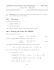### SD #23 Career Transitions Programs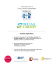### Orientation Determination from Cryo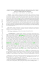### Library-based solutions for algorithms with complex patterns of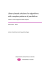### Our Brochure - Access Building Products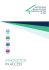### CCG-RUMBA • Core™ 2 Duo CompactPCI ® CPU### study guide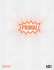### XRY 7.0 Release Notes EN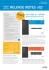### Optimizing Response Time, Rather than Hit Rates, of WWW Proxy